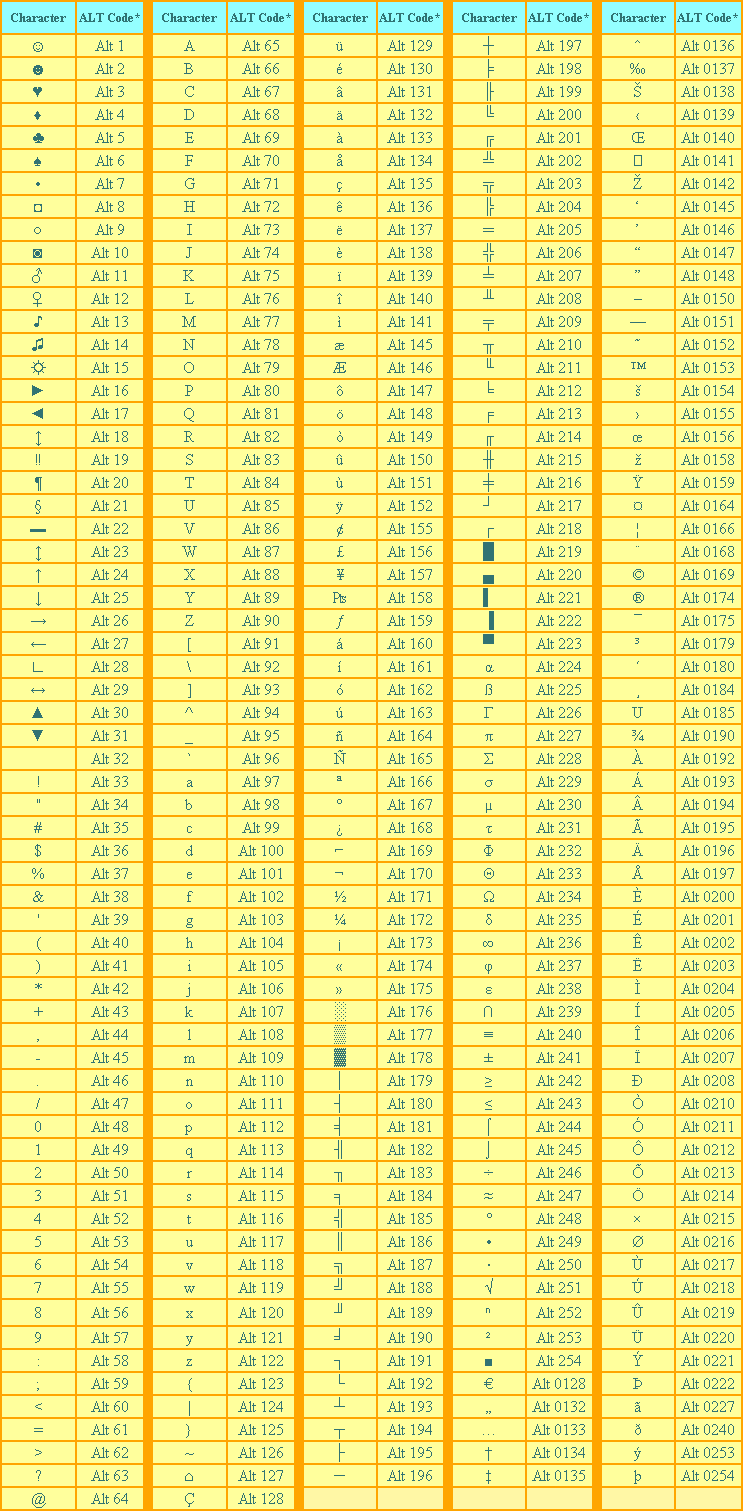Loading...

# ALT Codes

ALT Codes

I) Make certain that the Num Lock  key has been pressed

to activate the numeric key section of the keyboard.

II)

Depress the Alt key.

III) While the Alt key is depressed, type the proper sequence

of numbers (on the numeric keypad) of the ALT code from

the table below.

IV)
Release the Alt key, and the character will appear.

V) On a laptop computer, there usually is no numeric

keypad. So, instead, depress the Fn (function) key,

then the Alt key, then the numerical code sequence.

VI)
The numbers are on the fronts of certain keyboard keys:

M=0, J=1, K=2, L=3, U=4, I=5, O=6,  7=7, 8=8, and 9=9.